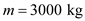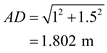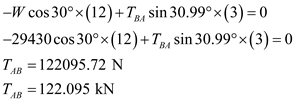# In the boring rig shown, the center of gravity of the .3000-kg tower is located at point G. For the position shown, determine the force exerted by the hydraulic cylinder AB.

Question-AnswerCategory: Engineering MechanicsIn the boring rig shown, the center of gravity of the .3000-kg tower is located at point G. For the position shown, determine the force exerted by the hydraulic cylinder AB.

In the boring rig shown, the center of gravity of the .3000-kg tower is located at point G. For the position shown, determine the force exerted by the hydraulic cylinder AB.Step: 1

Mass of the tower,Weight of the body,Free body diagram:Step: 2

From the diagram,Step: 3

Consider equilibrium equationsTherefore, the force exerted by the cylinder AB is